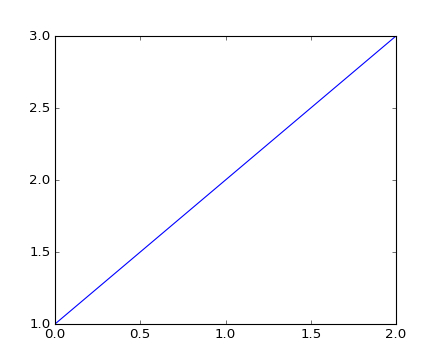### We're updating the default styles for Matplotlib 2.0

Learn what to expect in the new updatesTravis-CI:# pylab_examples example code: agg_buffer.py¶```#!/usr/bin/env python
"""
Use backend agg to access the figure canvas as an RGB string and then
convert it to an array and pass it to Pillow for rendering.
"""

import numpy as np

import matplotlib.pyplot as plt
from matplotlib.backends.backend_agg import FigureCanvasAgg

try:
from PIL import Image
except ImportError:
raise SystemExit("Pillow must be installed to run this example")

plt.plot([1, 2, 3])

canvas = plt.get_current_fig_manager().canvas

agg = canvas.switch_backends(FigureCanvasAgg)
agg.draw()
s = agg.tostring_rgb()

# get the width and the height to resize the matrix
l, b, w, h = agg.figure.bbox.bounds
w, h = int(w), int(h)

X = np.fromstring(s, np.uint8)
X.shape = h, w, 3

try:
im = Image.fromstring("RGB", (w, h), s)
except Exception:
im = Image.frombytes("RGB", (w, h), s)

# Uncomment this line to display the image using ImageMagick's
# `display` tool.
# im.show()
```

Keywords: python, matplotlib, pylab, example, codex (see Search examples)